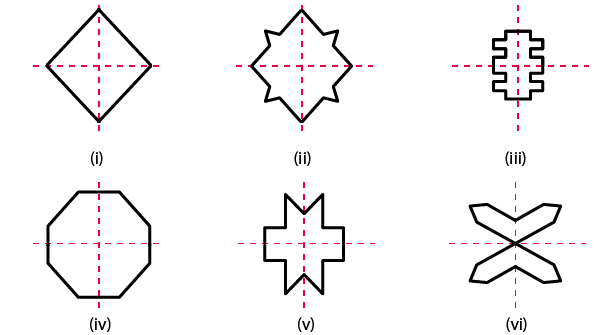# RD Sharma Solutions for Class 6 Maths Chapter 17: Symmetry Exercise 17.2

In this exercise, we discuss the construction of some two-dimensional symmetric figures as activities. We also get an idea about the concept of symmetrical figures with two lines of symmetry. The students can make use of these solutions created by experts having vast experience in the education industry. These solutions also contain various methods which can be followed in solving the complex problems in a shorter duration. To know more about the concepts which are explained here, students can download RD Sharma Solutions Class 6 Maths Chapter 17 Symmetry Exercise 17.2 PDF which are provided here.

## RD Sharma Solutions for Class 6 Maths Chapter 17: Symmetry Exercise 17.2 Download PDF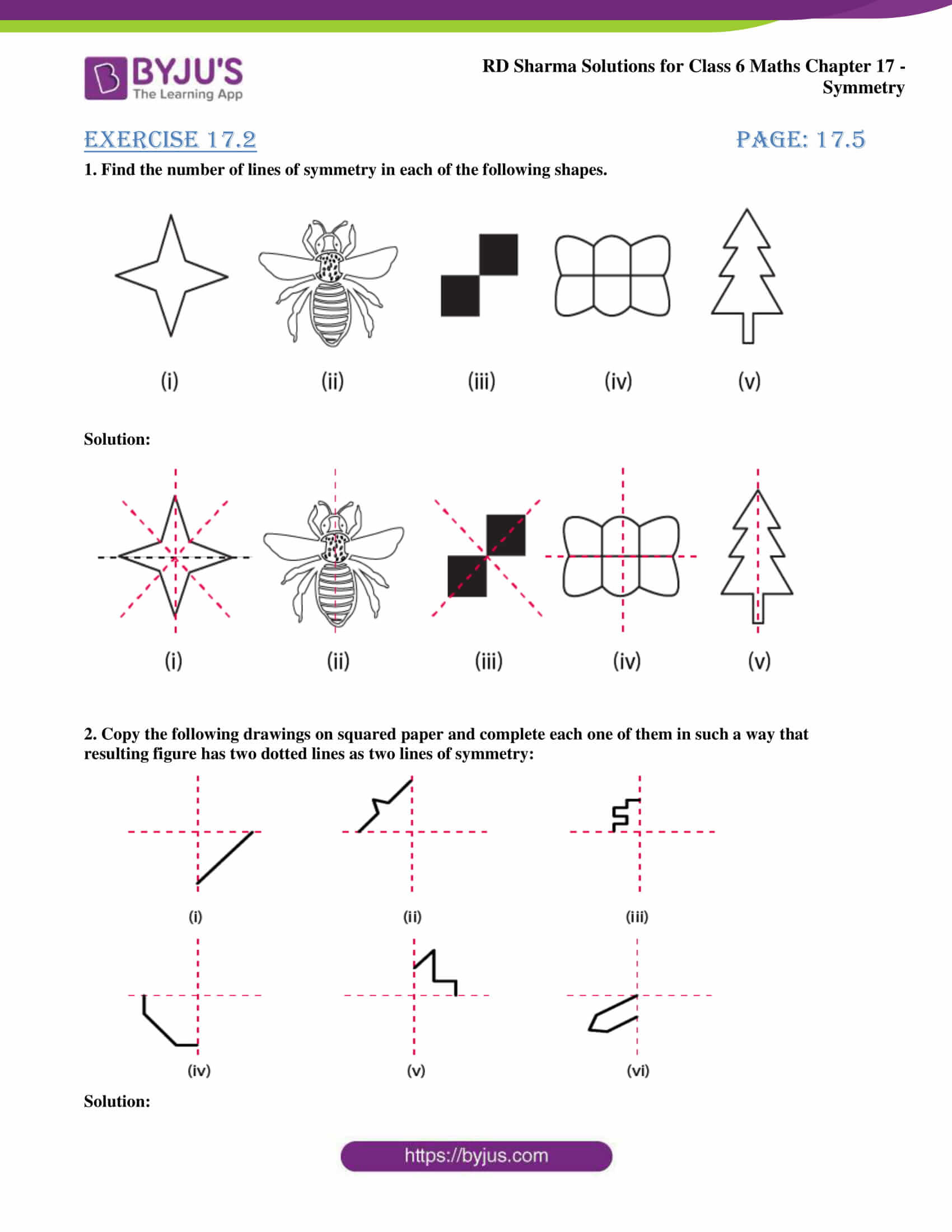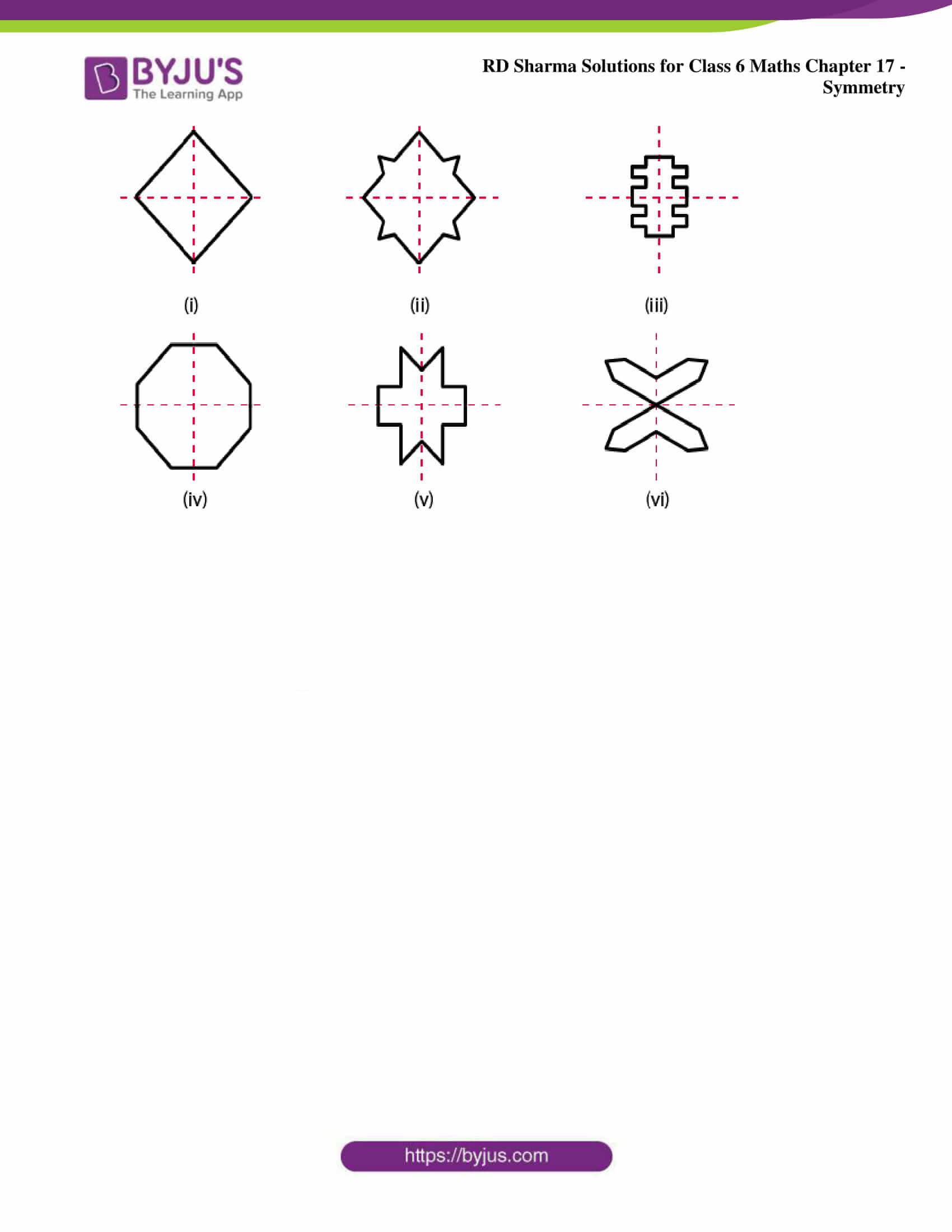### Exercise 17.2 page: 17.5

1. Find the number of lines of symmetry in each of the following shapes.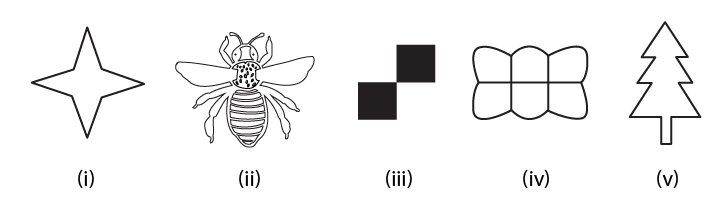Solution: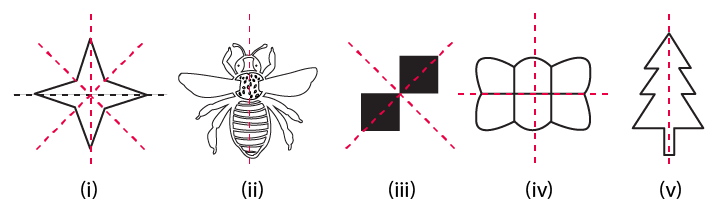2. Copy the following drawings on squared paper and complete each one of them in such a way that resulting figure has two dotted lines as two lines of symmetry: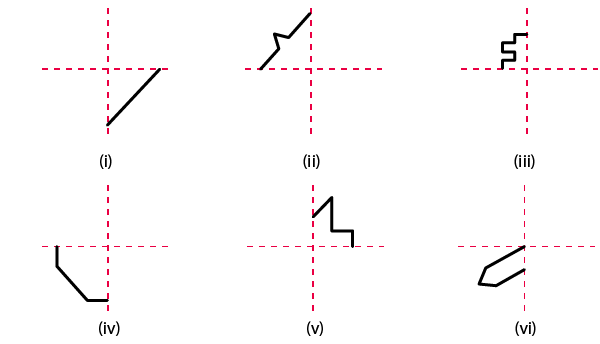Solution: